Resistor Circuits - Practice Exercises

` `
`Try each problem below - some practice exercises for you! `

Suggestion: First sketch a circuit diagram on a scrap of paper. Enter the information that you are given. Write down what you have to find too - the missing information. Then decide how the various Ohm's Law formulas can be used to find the solution.

Nominal "names" are given in these circuits to identify the current, the voltage and the resistors themselves.

To check your answer: Place your cursor over the antenna symbol alongside. The correct solution will appear! You don't have to click.

The SIMPLE circuit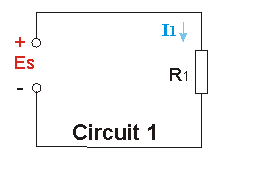A Given I1= 2 amp, and  R1 = 3 ohm,  find Es.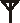B Given Es = 12 volt, R1 = 2 ohm,  find I1.C Given that Es = 100 volt, I1 = 50 mA, find R1.D Given that R1 = 10 ohm, I1 = 3 Amp, find the power dissipated in R1.E Given that R1 = 10 ohm, Es = 5 volt, find the power dissipated by R1.The PARALLEL circuit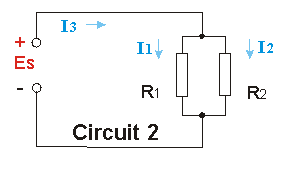F Given I1= 2 amp, and  I2 = 4 amp,  find I3.G Given Es = 12 volt, I3 = 2 amp, and that R1=R2, Find the value of each of  R1 and R2.H Given that R1 = 10 ohm, R2 = 10 ohm, and I3 = 2 amp, find Es.J Each resistor is 10 ohm, I3 is 6 amp, what is the power dissipated by each resistor?The SERIES circuit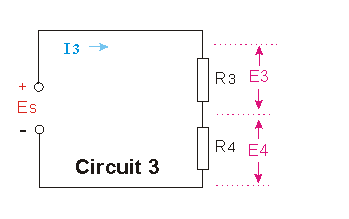K Given that R3=R4, and  Es = 12 volt,   find E3 and E4.L Given Es = 12 volt, I3 = 2 amp, and that R3=R4, Find the value of each of  R3 and R4.M Given that R3 = 10 ohm, R4 = 20 ohm, and I3 = 2 amp, find Es.N Each resistor is 10 ohm, I3 is 6 amp, what is the power dissipated by each resistor?SERIES and PARALLEL circuits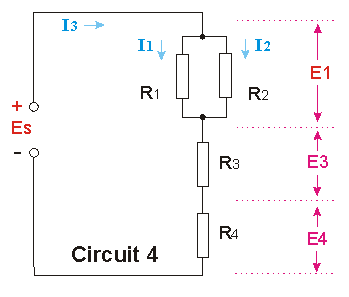O Given I1= 2 amp, and  I2 = 4 amp,  find I3.P Given that R1 = R2 = 8 ohm, I3 = 2 amp, Es = 12 volt,  find the total value of R3 and R4.Q Given that all resistors are 10 ohm, and I3 = 2 amp, find Es.R Each resistor is 10 ohm, I3 is 6 amp, what is E1, E3, E4 and Es?S Each resistor is 10 ohm, I3 is 6 amp, what is the power dissipated by each resistor?To top of page

__________________________________